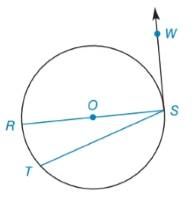Chapter 6.2, Problem 4EElementary Geometry For College St...

7th Edition
Alexander + 2 others
ISBN: 9781337614085

Solutions

Chapter
SectionElementary Geometry For College St...

7th Edition
Alexander + 2 others
ISBN: 9781337614085
Textbook Problem

Find m R T ⌢ if m ∠ R S T : m ∠ R S W = 1 : 5To determine

To find:

mRT if mRST:mRSW=1:5.

Explanation

Theorem:

The radius drawn to a tangent at the point of tangency is perpendicular to the tangent at that point.

Corollary:

The measure of an angle formed by a tangent and a chord drawn to the point of tangency is one-half the measure of the intercepted arc.

Calculation:

To find: mRSW.

Diameter is RS¯ and tangent is SW.

By theorem,

mRSW=90°

mRST:mRSW=1:5

mRSTmRSW=15mRSW=5mRST90°=5mRST

Still sussing out bartleby?

Check out a sample textbook solution.

See a sample solution

The Solution to Your Study Problems

Bartleby provides explanations to thousands of textbook problems written by our experts, many with advanced degrees!

Get Started

Simplify the expressions in Exercises 97106. (xy)1/3(yx)1/3

Finite Mathematics and Applied Calculus (MindTap Course List)

If x=1213 find the values of the other hyperbolic functions at x.

Single Variable Calculus: Early Transcendentals, Volume I

Using the power series for cos x, the sum of the series is: cos(0.0625) cos(0.25) cos(0.5)

Study Guide for Stewart's Single Variable Calculus: Early Transcendentals, 8th